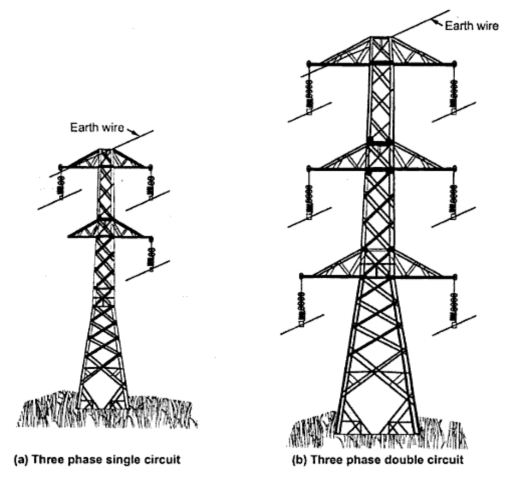### Methods of Arrangement Conductors

There are various methods of arranging the conductors over the line supports.
i) Single phase circuits :
There are two types of arrangements in single phase circuits viz single circuit and double circuit which are respectively shown in the Fig. 1(a) and (b). In double circuit there are again two subtypes of arrangements viz horizontal and vertical disposition.Fig. 1

1.1 Comparison of Single Circuit and Double Circuit
The comparison of single circuit and double circuit as given below
ii) Three phase circuits :
The three phase circuits are also having single circuit or double circuit arrangement. In single circuit arrangement of three phase circuit, the conductors are arranged either at the corners of an equilateral triangle or at the corners of right angled triangle for unequal distance between the conductors. The Fig. 2 shows various types of arrangements of three phase circuits.Fig. 2